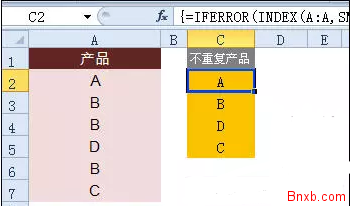EXCEL提取唯一值公式 提取不重复元素公式 INDEX公式应用

=IFERROR(INDEX(A:A,SMALL(IF(MATCH(A\$2:A\$7,A\$2:A\$7,0)=ROW(A\$1:A\$6),ROW(A\$2:A\$7)),ROW(A1))),"")• ^